### LOGARITHMS

Mathematics SSS 3 Second Term

WEEK 3

LOGARITHMS

Performance Objectives

Student should be able to:

1. Deduce the laws of logarithm.
2. Verify logarithms laws with simple exercises.
3. Use logarithm table to solve problems involving calculations

Content

Laws of indices

The following laws of indices are true for all non-zero values of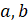and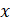.

1.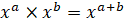2.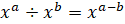3.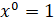4.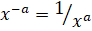5.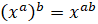6.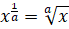7.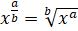or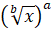8. .... View More